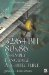Exercises1. What is an "inner product"?

2. A cross product is known by another name . What is it?

3. What happens to a vector if a negative scalar is applied as a product?

4. What is the solution for:

1. AB + C D, if A= 2, B = 5, C = 3, and D = 4?

2. A= B = C = D = 0x80000000?

3. With saturation?

4. Without saturation?

5. What is the equation for a dot product?

6. Given the two vertices v :{8, 4, 6, 4} and w :{8, 2, 6, 8}, resolve

1. v + w

2. vw

3. v w

4. v w32/64-Bit 80x86 Assembly Language Architecture
ISBN: 1598220020
EAN: 2147483647
Year: 2003
Pages: 191

Similar book on Amazon